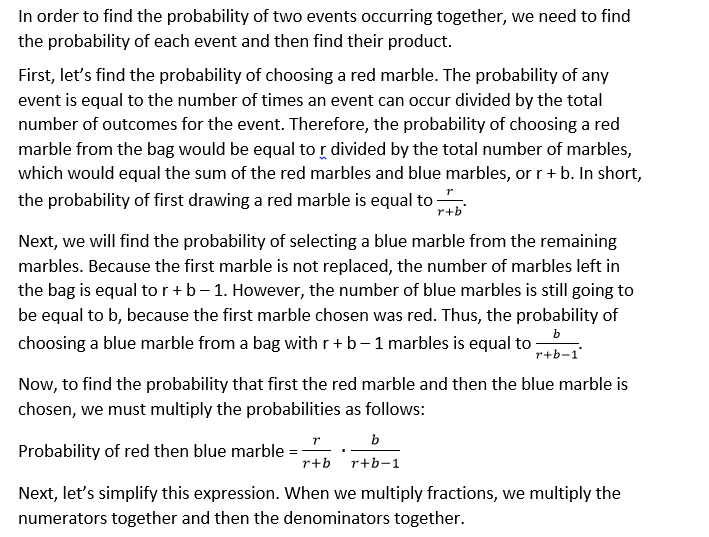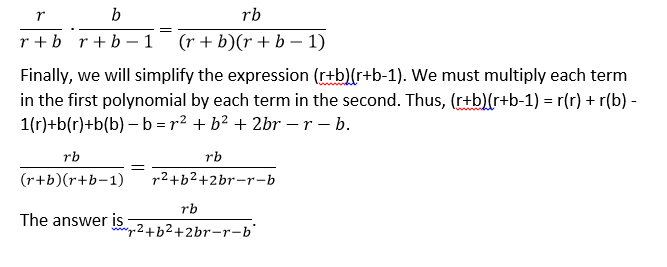# PSAT Math : How to find the probability of an outcome

## Example Questions

### Example Question #22 : How To Find The Probability Of An Outcome

A number between 1 and 15 is selected at random.  What are the odds the number selected is a multiple of 6?

3/7

3/15

1/7

2/15

2/15

Explanation:

In the set of 1 to 15, two numbers, 6 and 12, are multiples of 6. That means there are two chances out of 15 to select a multiple of 6.

2/15

### Example Question #23 : How To Find The Probability Of An Outcome

A big box of crayons contains a total of 120 crayons.

The box is composed of 3 colors; red, blue, and orange. 30 of the crayons are red, 40 of the crayons are blue and the rest are orange. If one picks a crayon randomly from the box, what is the probability that it will be orange?

3/7

5/12

2/7

1/3

7/12

5/12

Explanation:

To solve the problem one must calculate that there are 50 orange crayons in the box. So 50/120 are orange. If we simplify that fraction by 10 we get 5/12.

### Example Question #21 : How To Find The Probability Of An Outcome

A skydiver is trying to determine the probability of landing within the target of a grass field. If the field measures 1000 meters by 500 meters and the target area measures 50 meters by 50 meters, what is the probability of the skydiver landing in the target area?

1/100

3/400

1/200

3/200

5/200

1/200

Explanation:

Find the area of the entire field and the target area. The fraction of the field that is the target area is equal to the probability of the skydiver hitting the target area. For example, if the field were 100 m3 and the target area was 100 m3 than the probability would be 1. If the field were 100 m3 and the target area was 50 m3 than the probability would be 0.5 and so on.

(50 * 50)/(1000 * 500) = 5/1000 = 1/200

### Example Question #31 : Probability

If a container holds 4 red balls, 3 yellow balls, and 2 blue balls, what are the odds of picking out both of the blue balls without replacement?

1/72

1/6

1/8

2/9

1/36

1/36

Explanation:

You take the probability of the first outcome times the probability of the second, so (2/9) * (1/8) = 2/72 = 1/36

### Example Question #31 : How To Find The Probability Of An Outcome

Let R = {1, 3, 4, 12}, and let Q = {2, 6, 8, 24}. If one number is randomly selected from R, and another is randomly selected from Q, what is the probability that the product of those two randomly chosen numbers will belong to Q?

1/4

5/16

5/8

9/16

1/2

9/16

Explanation:

First, let's consider all of the pairs that could be chosen from R and Q. There are sixteen possibilities:

1 and 2; 1 and 6; 1 and 8; 1 and 24; 3 and 2; 3 and 6; 3 and 8; 3 and 24; 4 and 2; 4 and 6; 4 and 8; 4 and 24; 12 and 2; 12 and 6; 12 and 8; 12 and 24.

Now, we need to find the product of each of these pairs, and then determine whether or not they belong to Q. The products of all of the possible pairs would be as follows:

1(2) = 2, which belongs to Q

1(6) = 6, which belongs to Q

1(8) = 8, which belongs to Q

1(24) = 24, which belongs to Q

3(2) = 6, which belongs to Q

3(6) = 18, which doesn't belong to Q

3(8) = 24, which belongs to Q

3(24) = 72, which doesn't belong

4(2) = 8, which belongs to Q

4(6) = 24, which belongs to Q

4(8) = 32, which doesn't belong

4(24) = 96, which doesn't belong

12(2) = 24, which belongs to Q

12(6) = 72, which doesn't belong

12(8) = 96, which doesn't belong

12(24) = 288, which doesn't belong

Thus, of our sixteen possible combinations, there are 9 which, when multiplied, give a number that belongs to Q. Because probability is the number of successful events out of the total number of events, the probability is 9/16.

### Example Question #191 : Data Analysis

1) If John does not have freckles, he is not Tim's sibling.

2) If Jake does not have freckles, he is Tim's sibling.

3) If Suzy is not Tim's sibling, she will not have freckles.

4) All of the other answers are false.

All of Tim's siblings have freckles. Which of the above statements must be true?

3) If Suzy is not Tim's sibling, she will not have freckles.

1) If John does not have freckles, he is not Tim's sibling.

2) If Jake does not have freckles, he is Tim's sibling.

4) All of the other answers are false.

1) If John does not have freckles, he is not Tim's sibling.

Explanation:

Only John's statement must be true. Suzy could have freckles and not be Tim's sibling and if Jake doesn't have freckles then he is not Tim's sibling.

### Example Question #31 : How To Find The Probability Of An Outcome

A bag contains red marbles and blue marbles only. Let r equal the number of red marbles and b equal the number of blue marbles in the bag. Mark draws one marble from the bag and then a second one without replacing the first. Which of the following expressions is equivalent to the probability that Mark will draw a red marble and then a blue marble?

(rb – r – b)/(r+ b+ 2br – r – b)

(r + b – 1)/(r+ 2br + b2)

rb/(r+ 2br + b2)

rb/(r+ b2)

rb/(r+ b+ 2br – r – b)

rb/(r+ b+ 2br – r – b)

Explanation:### Example Question #31 : Probability

In a bag, there are 5 blue marbles, 3 red marbles, and 2 green marbles.  If three marbles are chosen consecutively at random without replacement, what is the probability that the color of the chosen marbles would be blue-red-green in that order?

1/300

1/3

3/100

1/1000

1/24

1/24

Explanation:

The probability that a blue marble is chosen first is 5/10 because at the outset there are 5 blue marbles in a total of 10 marbles.  After one marble has been chosen, there are 9 total marbles remaining since there is no replacement.  The probability that a red marble is chosen in the second pick is 3/9 (because there are 3 red marbles out of a total of 9).  There are now 8 total marbles remaining and 2 green marbles. Thus, the probability of picking a green marble on the third pick is 2/8.

Therefore, the probability of picking a blue-red-green marble outcome (in that order) is:

5/10 * 3/9 * 2/8 = 30/720 = 1/24

### Example Question #31 : Probability

A book shelf has 15 theology books, 20 philosophy books, and 5 history books. If someone were to draw two texts at random, what is the chance that they would draw at least one theology book?

5/13

44/121

9/64

8/13

8/13

Explanation:

Thinking through our data, we know that we are looking for the following combination of events, where T is a theology book, and < > represents a draw from the shelf:

1. <T><T>

2. <T><Non-T>

3. <Non-T><T>

To save us quite a bit of trouble, let us note that only one event is excluded:

<Non-T><Non-T

The easiest way to solve this would be to solve for the probability of this one case and subtract that from 1. This will give us the "remaining probability" that applies to the three cases that we want.

The <Non-T><Non-T> case would be calculated:

First draw: 25/40 = 5/8

Second draw: 24/39 = 8/13

Total probability: (5/8) * (8/13) = 5/13

The probability of our case is 1 – (5/13) = (13 – 5)/13 = 8/13.

### Example Question #31 : Probability

A drawing is being held for concert tickets at a high school. There are 200 seniors, 150 juniors, 200 sophomores and 150 freshmen. Each senior's name is placed in the drawing 4 times, each junior's 3, sophomores 2, and freshmen one time. What is the probability that a senior's name will be chosen?

2/9

2/7

4/9

5/9

2/9